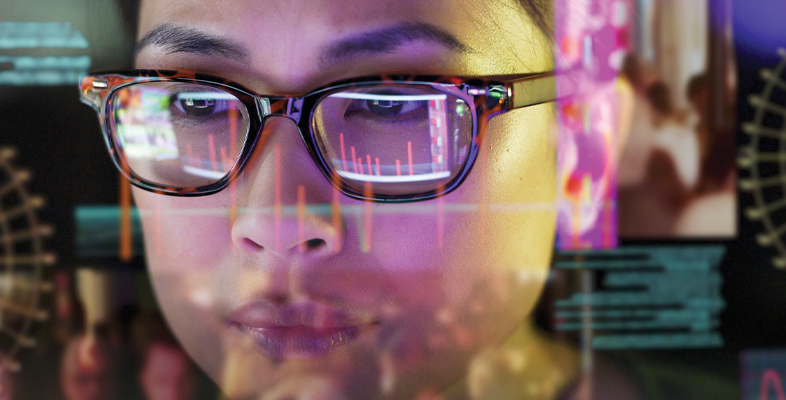Digital thinking tools for better decision making

Start this free course now. Just create an account and sign in. Enrol and complete the course for a free statement of participation or digital badge if available.

Free course

# 5 Testing, testing

Suppose that one person in every thousand is known to have a fatal infection. (This is called the base rate.) Scientists have developed a test for the disease and experiments have shown that the test is 99% accurate.

By 99% accurate we mean that, on average, 99 out of every 100 people who test positive will have the disease, and 99 out of every 100 who test negative will not have the disease.

As part of a routine health check, you are found to test positive.

## Activity 6 Take a guess

Timing: Allow 1 minute.

Given the information above, you want to know what the chances are that you have the disease. Which of the following is the best answer?

• A.99%
• B.90%
• D.1 %

### Discussion

The correct answer is C, about 9%. There is a greater than 90% chance that you don’t have the disease!

Most people overestimate the chances, because it is natural to focus on the fact that the test is 99% accurate. In a study reported in 2008 (Gigerenzer et al.), the majority of a group of 160 doctors who were asked a similar question gave the wrong answer.

To see why the answer to Activity 6 is 9%, it helps to do a thought experiment with a concrete number of people. A good choice is 100000, because everything works in whole numbers.

Figure 19 shows a whole population of 100000 split into two groups: those who have the disease (D) and those who do not (ND). Because 1 per thousand has the disease, on average 100 have the disease and the other 99 900 don’t.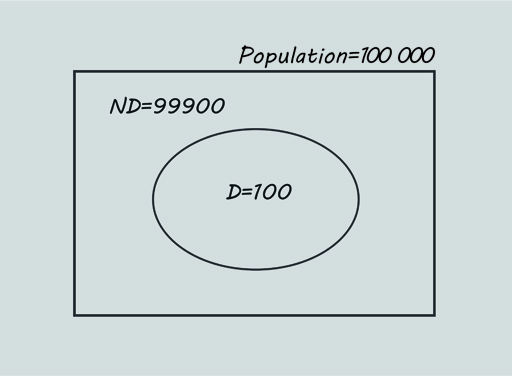Figure 21 Population split into D and ND

First, consider set D. The accuracy is 99%, meaning that 99 of the 100 with the disease will test positive (D+) and 1 will test negative (D-), as shown in Figure 20.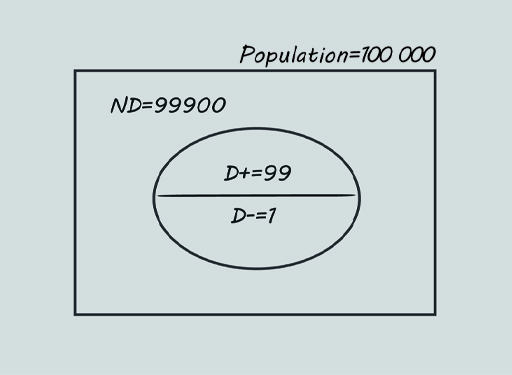Figure 22 D is split into D+ and D-

Next, consider set ND.

Because the accuracy is 99%, that means 99% of the 99900 who don’t have the disease will test negative. This comes to 98901 people. The remaining 1% of ND (999 people) will test positive. These numbers are shown in Figure 21.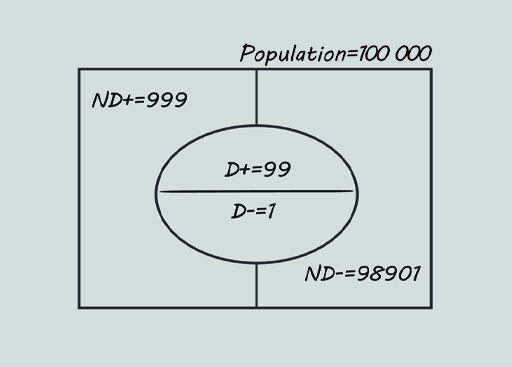Figure 23 ND is split into ND+ and ND-

You tested positive, so focus on the positives (Figure 22).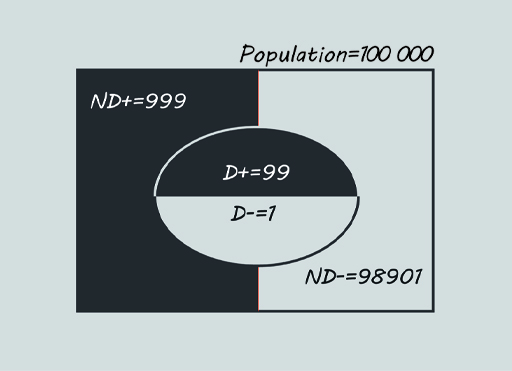Figure 24 Of 999 + 99 positives, only 99 have the disease

There are 999 + 99 = 1098 testing positive altogether, but only 99 of these actually have the disease. So the probability that someone testing positive is actually infected is

99/1098 = 9.0% to one decimal place.

The probability that they are not infected is thus 100% - 9% = 91%. Your chances are good!

Hopefully this explanation has convinced you of the correct answer and, if you were given a different base rate (or a different test accuracy), you could follow a parallel set of calculations and find the new probability of a person testing positive being infected.

However, it’s possible to capture the details of the calculation in a single formula. This doesn’t involve the size of the population, which was just a number chosen for convenience. If a different one, 1 000 000 say, had been used instead, the final answer would have ended up the same.

Using brt for the base rate of the disease, and acc for the accuracy, expressed as decimal fractions, e.g. 0.001 and 0.99, the formula for the probability, when written in Python is

brt*acc / (brt*acc + (1 – brt)*(1 – acc))

In this formula

brt*acc represents D+, the proportion of the population who have the disease and test positive

(1 – brt)*(1 – acc)) represents ND+, the proportion of the population who do not have the disease and test positive, and therefore

(brt*acc + (1 – brt)*(1 – acc))

represents the total proportion testing positive, and the division is working out

That is, the chances that a person who tests positive is actually infected.

You can try this for yourself in the next activity.

## Activity 7 Playing in the sandpit

Timing: Allow 5 minutes

The following shows how the example you saw worked out with sets could be done in the Python shell, using the formula.

>>> brt = 0.001

>>> acc = 0.99

>>> brt*acc / (brt*acc + (1 - brt)*(1 - acc))

0.09016393442622944

You do not need to type in the expression

brt*acc / (brt*acc + (1 - brt)*(1 - acc))

You can copy it from here and paste it in at the prompt >>>.

Active content not displayed. This content requires JavaScript to be enabled.
Interactive feature not available in single page view (see it in standard view).

To get the result as a tidy percentage, use

>>> round(_*100, 1)

9.0

1. Try this in the Python shell now and check that you get the correct answer, 9.0.
2. Now repeat the calculation but begin by leaving the accuracy the same but setting the base rate to
• 0.01 (i.e. 1 in 100)
• 0.1 (i.e. 1 in 10)

Observe the effect on the probability of being infected.

### Discussion

The probabilities are now 50.0% and 91.7%, respectively. As the base rate gets bigger, the probability becomes more like the figure intuitively expected.

The effect you have been exploring is often called the false positive paradox. It arises whenever the base rate is small compared with the rate of false positives, so the number of actual cases is swamped by the number of false positives. However, people don’t tend to take the base rate into account. The figure that stands out is the accuracy of 99%.

Consequently, when the base rate is low, as is usually the case, people grossly overestimate the likelihood that a positive test result is conclusive.

DTT_1